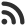Feed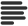Articles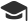Tutorials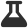Lab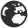CompaniesLeaderboard
 DG Student at BIT Mesra Aug. 30, 2020, 11:07 a.m. ⋅ 553 views

# DXC Placement Code Snippets Output Based Questions with answers

1. What will be the output of following code :

``````int main()
{
int a = NULL – true;
printf(“%d”,a);
return 0;
}``````

A. -1
B. Garbage value
C. 0
D. Error

Ans. -1

2. What will be the output of following code :

``````main()
{

extern int iExtern;
iExtern = 20;
printf(%d,iExtern);

}``````

A. 2
B. 20
C. Error
D. Warning

Ans. C

3. What will be the output of following code :

``````int x = 0;

int main()
{

if(x == x)
printf(“if”);
else
printf(“else”);
return 0;

}``````

A. Two same variables can not be compared
B. ifelse
C. else
D. if

Ans D

4. What will be the output of following code :

``````#define FALSE -1

#define NULL 0

#define TRUE 1

int main()
{
if(NULL)
printf(“NULL”);
else if(FALSE)
printf(“TRUE”);
else
printf(“FALSE”);
return 0;
}``````

A. TRUE
B. FALSE
C. NULL
D. Error

Ans. A

5. What will be the output of following code :

``````int main()
{

int i;
if(true)
printf(“work”);
else
printf(“not work”);
return 0;

}
``````

A. work
B. not work
C. compiler error
D. runtime error

Ans. A

6. What will be the output of following code :

``````int main()
{
if(printf(“0”))
printf(“inside if block”);
else
printf(“inside else block”);
return 0;

}``````

A. inside else block
B. 0
C. 0inside if block
D. Error – If can not have print statement

Ans. C

7. What will be the output of following code :

``````#define clrscr() 100;

main()
{

clrscr();
printf(%d\n\t, clrscr());

}``````

A. 100
B. 10
C. Compile Error
D. Runtime Error

Ans. A

8. What will be the output of following code :

``````int main()
{
void vpointer;

char cHar = g,cHarpointer = GOOGLE;

int j = 40;

vpointer = &cHar;

printf(“%c”,(char)vpointer);

vpointer = &j;

printf(“%d”,(int )vpointer);

vpointer = cHarpointer;

printf(“%s”,(char)vpointer +3);

}``````

A. 40
B. 100
C. ERROR
D. Warning

Ans. C

9. What will be the output of following code :

``````int main()
{

int i = 5, j = 4;

if(!printf(“”))
printf(“%d %d”, i, j);
else
printf(“%d %d”, i++, ++j);
return 0;

}``````

A. 5 5
B. 5 4
C. 5 6
D. 6 6

Ans. B

10. What will be the output of following code :

``````main()
{

int i = 10, j =20;
j = i ,j?(i,j)?i :j:j;
printf(“%d %d”,i,j);

}``````

A. 20 20
B. 10 10
C. 10 20
D. 10 10

Ans. D

Events

Nov. 28, 2018, 5:30 p.m.

Python from zero to hero

place Delhi

Aug. 13, 2018, 5:30 p.m.

Python from zero to hero

place Bangalore ( HackersFriend office BTM Layout)